“CBAM: Convolutional Block Attention Module” proposes a simple and effective attention module for CNN which can be seen as descendant of Sqeeze and Excitation Network. It will be presented on ECCV2018 and now available on Arxiv. The authors are Sanghyun Woo, Jongchan Park, Joon-Young Lee, and In So Kweon from KAIST, Lunit, and Adobe Research.

# Summary

• Research Objective
• The goal is to increase representation power by using attention mechanism: focusing on important features and supressing unnecessary ones.
• Proposed Solution
• Propose Convolutional Block Attention Module (CBAM), a simple and effective attention module for feed-forward convolutional neural networks.
• Given an intermediate feature map, the module sequentially infers attention maps along two separate dimensions, channel and spatial, then the attention maps are multiplied to the input feature map for adaptive feature refinement.
• Contribution
• Propose simple and effective attention module (CBAM) that can be widely applied to boost representation power of CNNs
• Validate the effectiveness of the attention module through extensive ablation studies
• Verify that performance of various networks is greatly improved on the multiple benchmarks by plugging the CBAM

# Convolutional Block Attention Module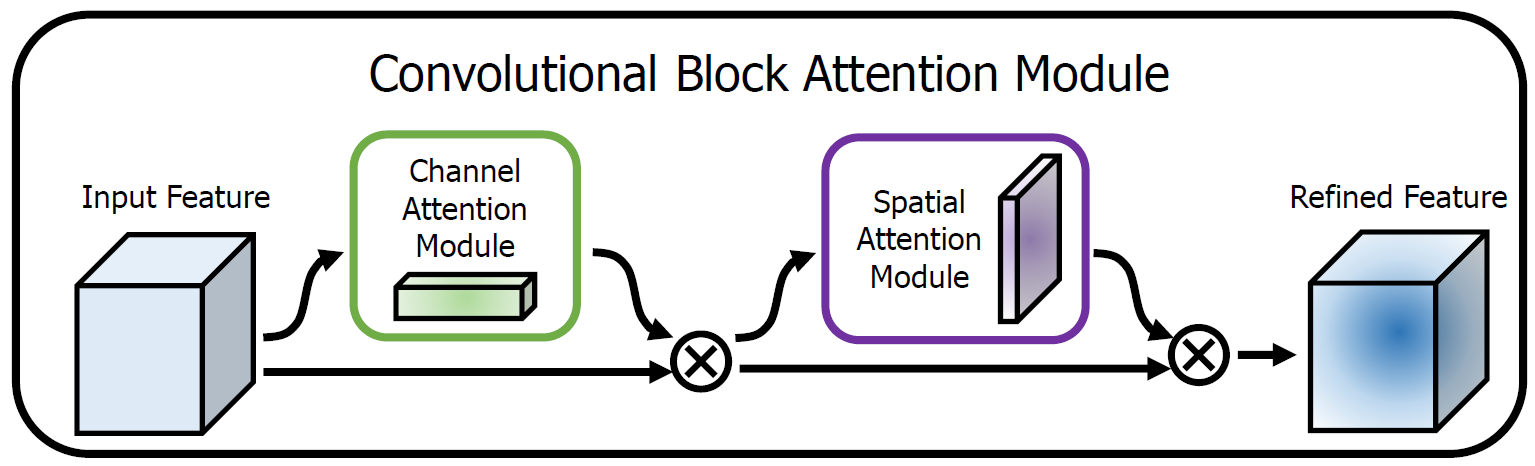Figure 1: The overview of CBAM. The module has two sequential sub-modules: channel and spatial. The intermediate feature map is adaptively refined through our module (CBAM) at every convolutional block of deep networks.

The overall attention process can be summarized as:

• Given an intermediate feature map $F \in \mathbb{R}^{C \times H \times W}$ as input
• CBAM sequentially infers a 1D channel attention map $M_c \in \mathbb{R}^{C \times 1 \times 1}$
• And a 2D spatial attention map $M_s \in \mathbb{R}^{1 \times H \times W}$
• $\otimes$: element-wise multiplication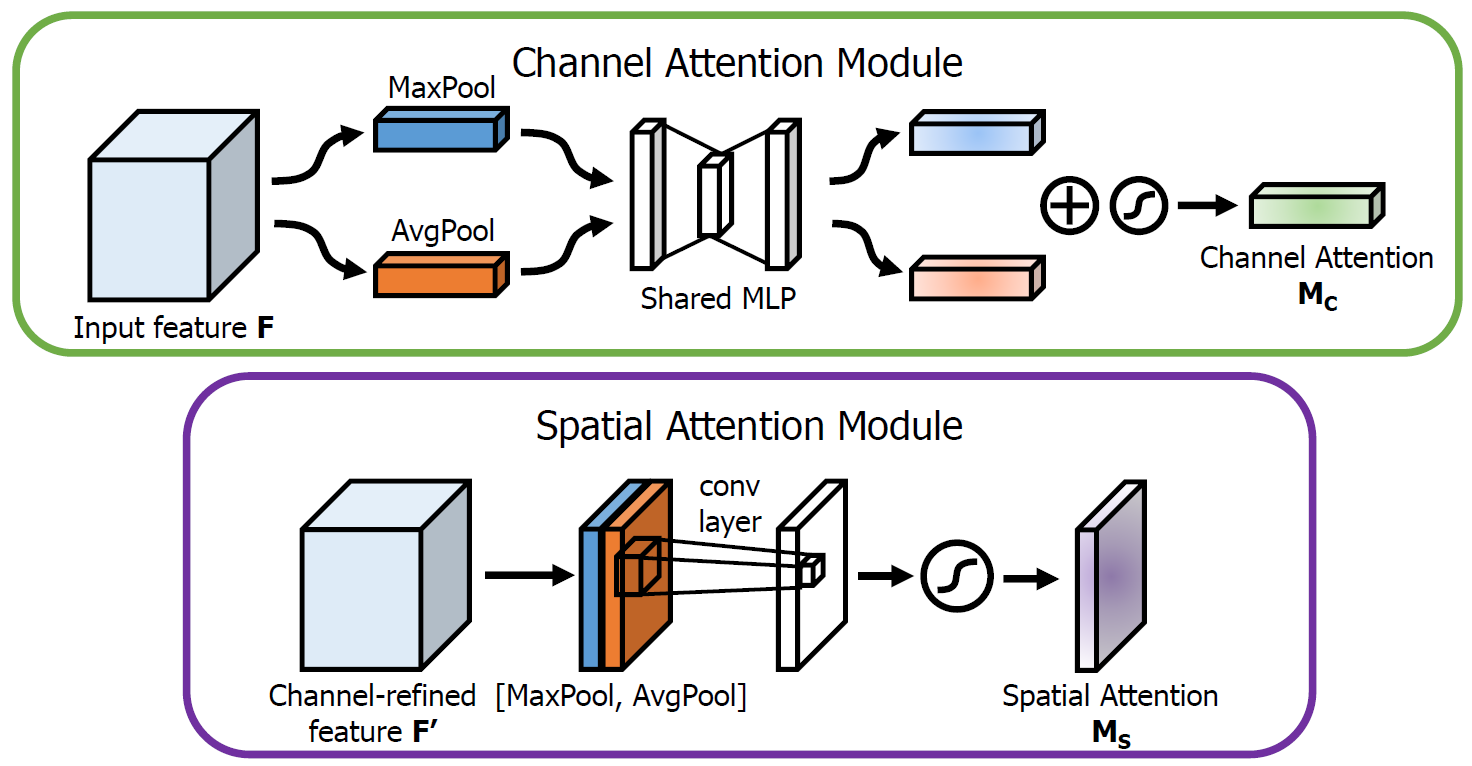Figure 2: Diagram of each attention sub-module. As illustrated, the channel sub-module utilizes both max-pooling outputs and average-pooling outputs with a shared network; the spatial sub-module utilizes similar two outputs that are pooled along the channel axis and forward them to a convolution layer.

## Channel Attention Module

The author produce a channel attention map by exploiting the inter-channel relationship of features. As each channel of a feature map is considered as a feature detector, channel attention focuses on ‘what’ is meaningful given an input image.

To compute the channel attention, we squeeze the spatial dimension of the input feature map. For aggregating saptial information, this paper use both average-pooled and max-pooled features simultaneously.

• Average pooling feature $F^c_{avg}$: learn the extent of the target object effectively and compute spatial statistics
• Max pooling feature $F^c_{max}$: gather distinctive object features to infer finer channel-wise attention

The channel attention is computed as:

• $sigma$: sigmoid function
• $W_0 \in \mathbb{R}^{C/r\times C}$ and $W_1 \in \mathbb{R}^{C\times C/r}$
• To reduce parameter overhead, the hidden activation size is set to $\mathbb{R}^{C/r\times 1 \times 1}$
• The MLP weights $W_0$ and $W_1$ are shared for both inputs and the ReLU activation function is followed by $W_0$

## Spatial Attention Module

The author generate a spatial attention map by utilizing the inter-spatial relationship of features. The spatial attention focuses on ‘where’ is an informative part.

On the concatenated feature descriptor, CBAM apply a convolution layer to generate a spatial attention map $M_s(F) \in \mathbb{R}^{H \times W}$ which encodes where to emphasize or suppress.

The spatial attention is computed as:

• Generated two 2D maps $F^s_{avg} \in \mathbb{R}^{1 \times H \times W}$ and $F^s_{max} \in \mathbb{R}^{1 \times H \times W}$ are concatenated and convolved by a standard convolution layer, producing the 2D spatial attention map.
• $f^{7\times 7}$: convolution operation with the filter size of $7 \times 7$

## Arrangement of Attention Modules

The author found that the sequential arrangement of two atention modules gives a better result than a parallel arrangement. And they shows that the channel-first order is slightly better than the spatial-first.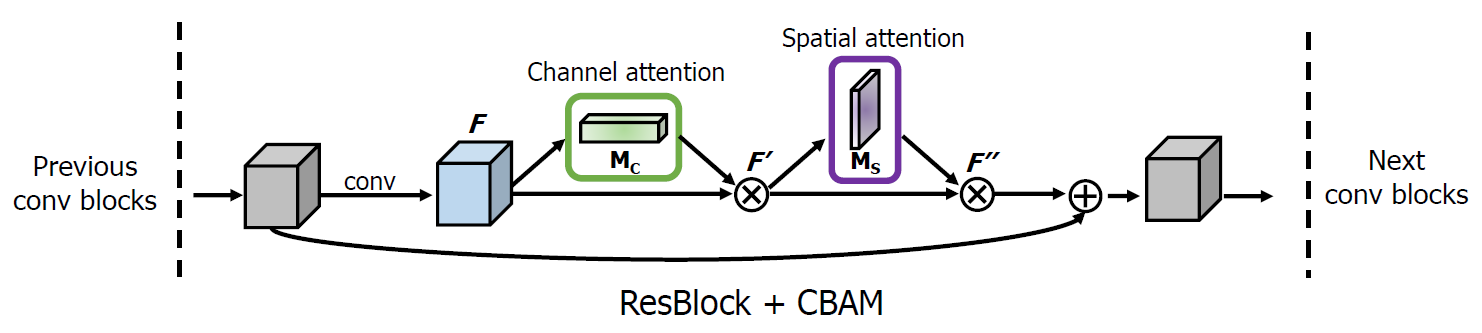Figure 3: CBAM integrated with a ResBlock in ResNet. This figure shows the exact position of the module when integrated within a ResBlock. We apply CBAM on the convolution outputs in each block.

# Experiment

## Ablation Studies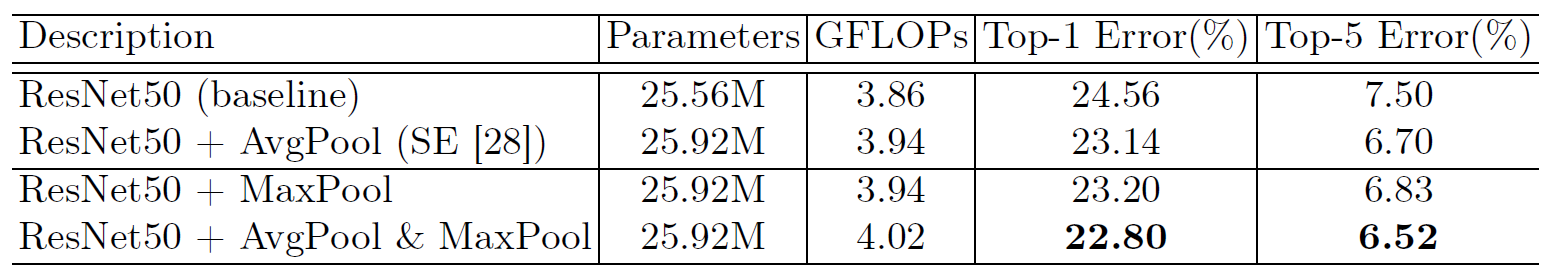Figure 4: Comparison of different channel attention methods. It shows that using proposed method outperforms recently suggested Squeeze and Excitation method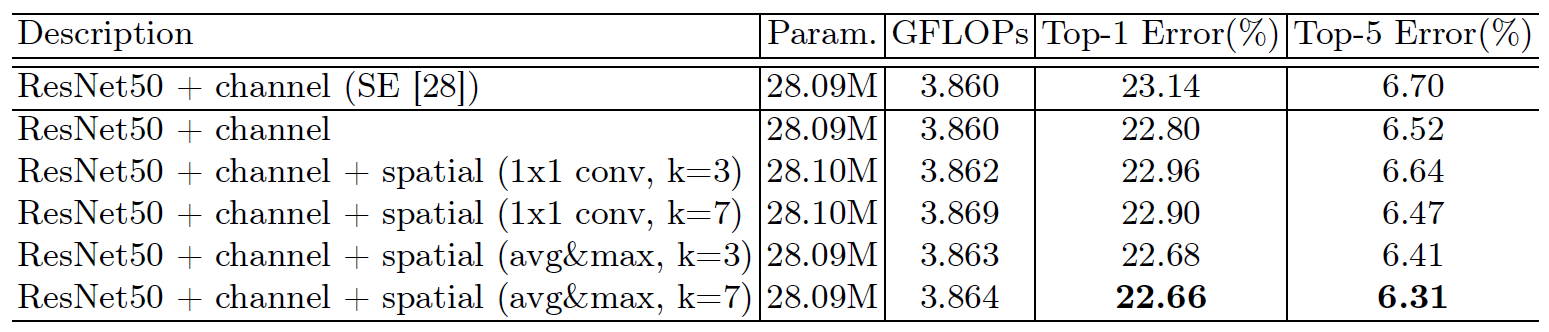Figure 5: Comparison of different spatial attention methods. Using the proposed channel-pooling (i.e. average- and max-pooling along the channel axis) along with the large kernel size of 7 for the following convolution operation performs best.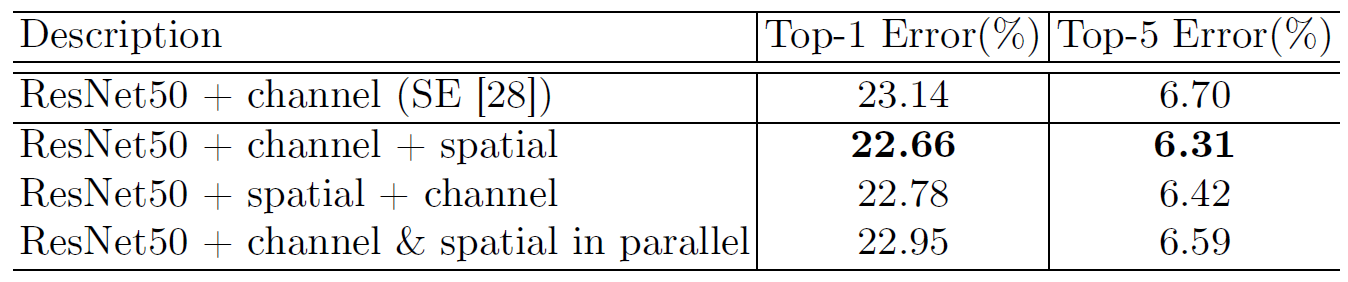Figure 6: Combining methods of channel and spatial attention. Using both attention is critical while the best-combining strategy (i.e. sequential, channelfirst) further improves the accuracy.

## ImageNet-1K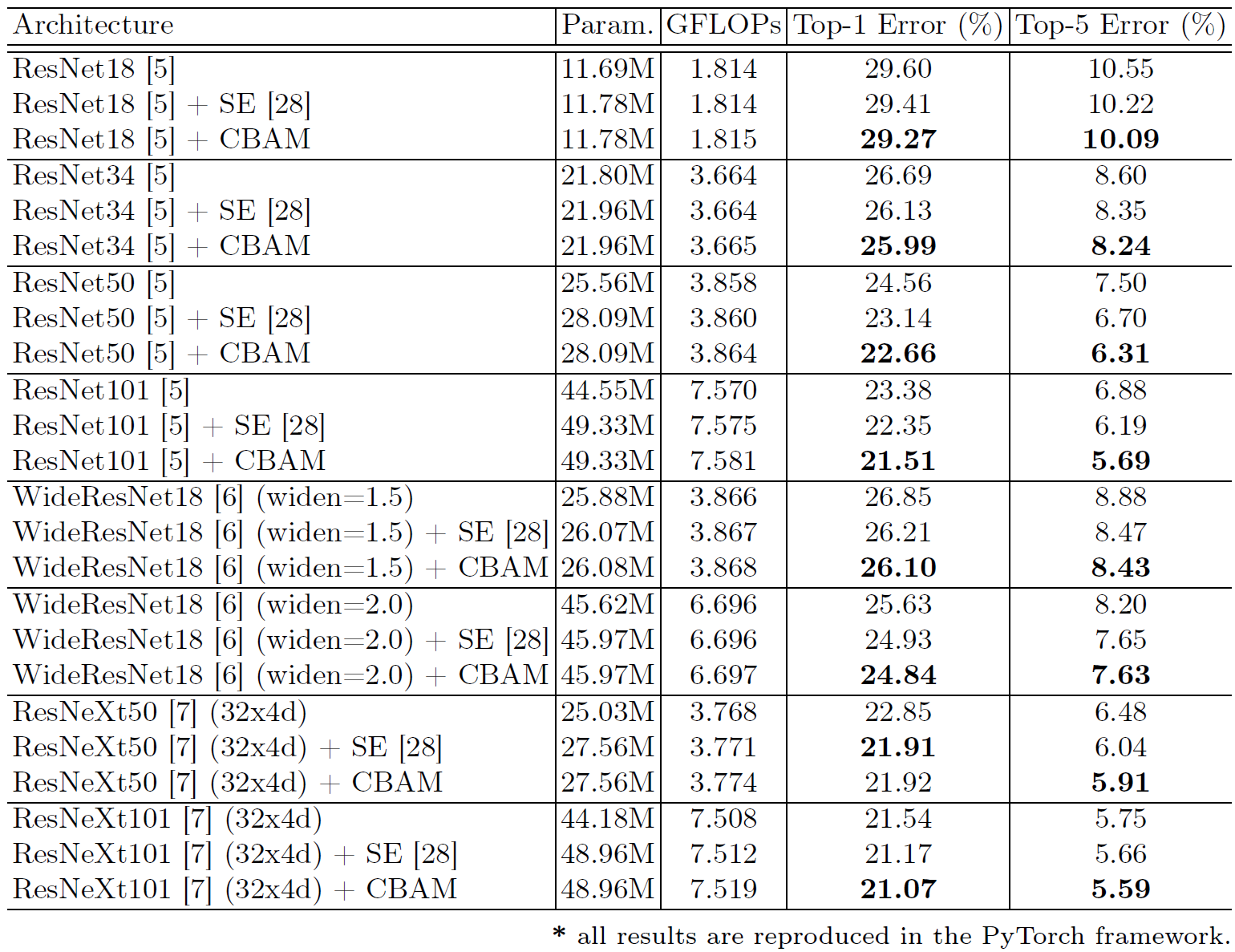Figure 7: Classification results on ImageNet-1K. Single-crop validation errors are reported.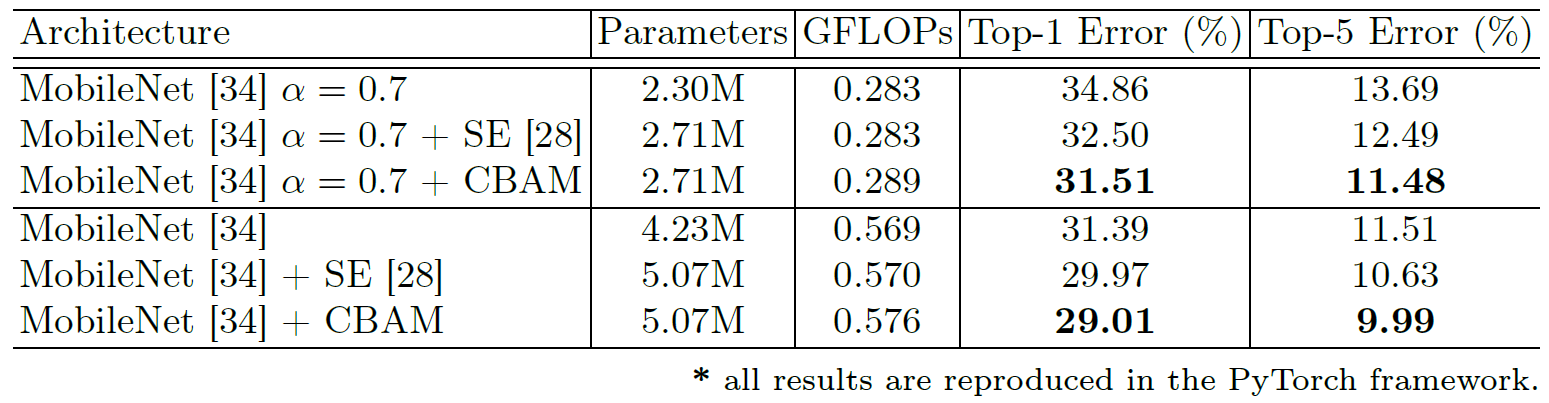Figure 8: Classification results on ImageNet-1K using the light-weight network, MobileNet. Single-crop validation errors are reported.

## Network Visualization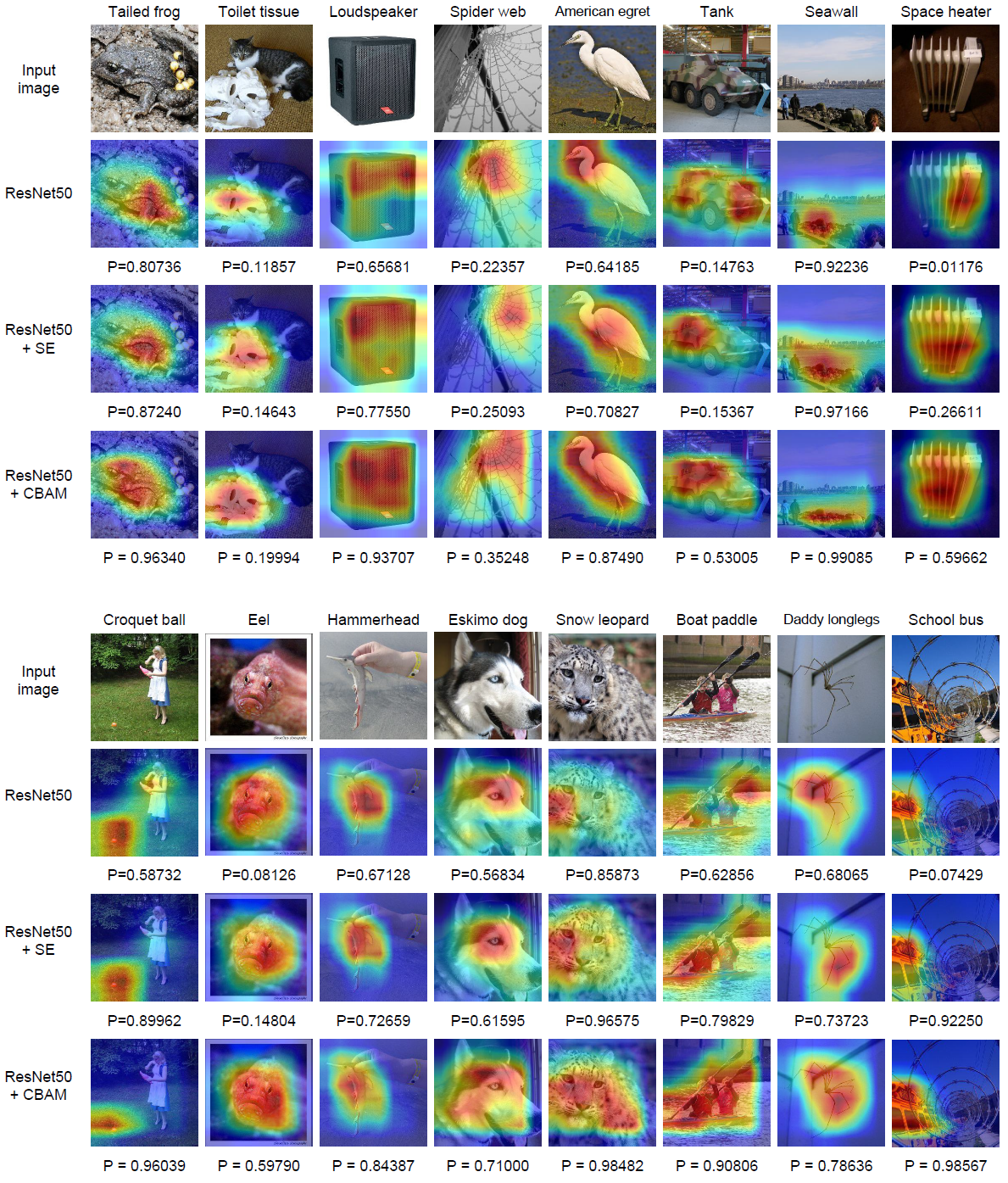Figure 9: Grad-CAM visualization results. We compare the visualization results of CBAM-integrated network (ResNet50 + CBAM) with baseline (ResNet50) and SE-integrated network (ResNet50 + SE). The grad-CAM visualization is calculated for the last convolutional outputs. The ground-truth label is shown on the top of each input image and P denotes the softmax score of each network for the ground-truth class.

## Object Detection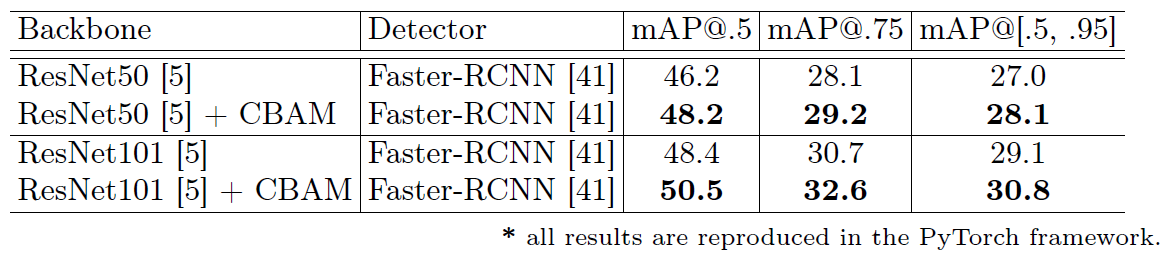Figure 10: Object detection mAP(%) on the MS COCO validation set. We adopt the Faster R-CNN detection framework and apply our module to the base networks. CBAM boosts mAP@[.5, .95] by 0.9 for both baseline networks.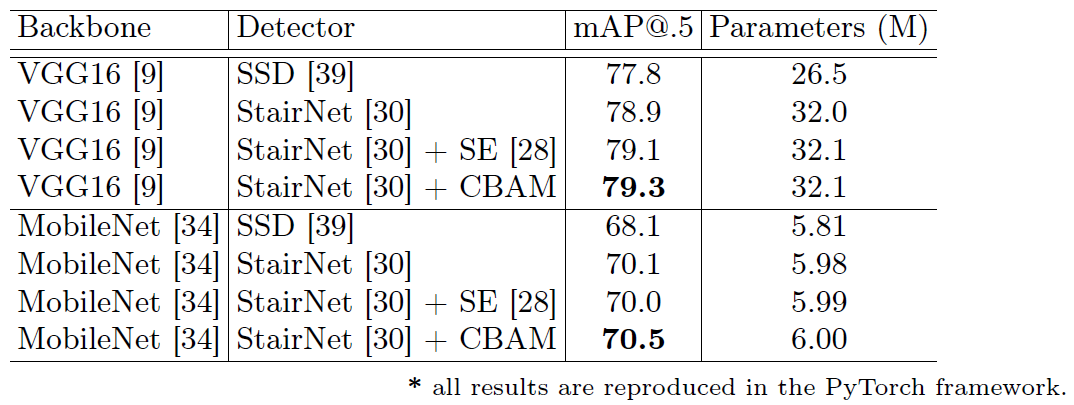Figure 11: Object detection mAP(%) on the VOC 2007 test set. We adopt the StairNet detection framework and apply SE and CBAM to the detectors. CBAM favorably improves all the strong baselines with negligible additional parameters.